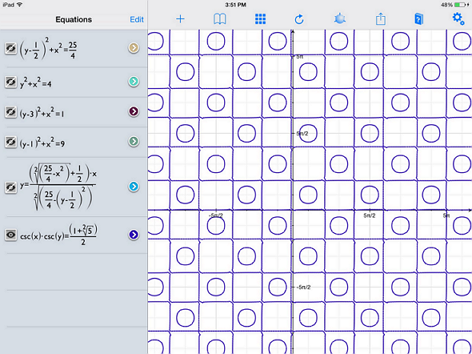# Checkers, Anyone?

Calculus Level 3The graph of $\csc(x)\csc(y)=\phi$ forms a bunch of squares and circles. The area of one of these circles is most nearly which of the following?

Remark: $\phi = \frac{1+\sqrt{5}}{2}$

×

Problem Loading...

Note Loading...

Set Loading...